×#### Thank you for registering.

One of our academic counsellors will contact you within 1 working day.

Click to Chat

1800-1023-196

+91-120-4616500

CART 0

• 0

MY CART (5)

Use Coupon: CART20 and get 20% off on all online Study Material

ITEM
DETAILS
MRP
DISCOUNT
FINAL PRICE
Total Price: Rs.

There are no items in this cart.
Continue Shopping• Complete JEE Main/Advanced Course and Test Series
• OFFERED PRICE: Rs. 15,900
• View Details

```Chapter 20: Surface Area And Volume of A Right Circular Cone Exercise – 20.1

Question: 1

Find the curved surface area of a cone, if its slant height is 60 cm and the radius of its base is 21 cm.

Solution:

It is given that:

Slant height of cone (l) = 60 cm

Radius of the base of the cone(r) = 21 cm

Curved Surface Area (C. S. A) = ?

C.S.A = πrl = 22/7 * 21 * 60 = 3960 cm2

Therefore the curved surface area of the right circular cone is 3960 cm2

Question: 2

The radius of a cone is 5cm and vertical height is 12 cm. Find the area of the curved surface.

Solution:

It is given that:

Radius of cone (r) = 5 cm

Height of the tent (h) = 12 cm

Slant Height of tent (l) = ?

Curved Surface Area (C. S. A) = ?

Now we know we that,

l = r2 + h2

l2 = r2 + h2

l2 = 52 + 122

l2 = 25 + 144

⟹ l = 13 cm

Now,

C.S.A = πrl

= 3.14 * 5 * 12

= 204.28 cm2

Therefore the curved surface area of the cone is 204.28 cm2

Question: 3

The radius of a cone is 7 cm and area of curved surface is 176 cm2. Find the slant height.

Solution:

It is given that:

Radius of cone(r) = 7 cm

Curved Surface Area (C. S. A) = 176 cm2

Slant Height of tent (l) = ?

Now we know,

C.S.A = πrl

⟹ πrl = 176

⟹ 22/7 ∗ 7 ∗ l = 176Therefore the slant height of the cone is 8 cm.

Question: 4

The height of a cone 21 cm. Find the area of the base if the slant height is 28 cm.

Solution:

It is given that:

Height of the traffic cone (h) = 21 cm

Slant height of the traffic cone (l) = 28 cm

Now we know that,

l2 = r2 + h2

282 = r2 + 212

r2 = 282 − 212

r = √77 cm

Area of the circular base = πr2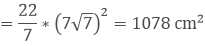Therefore the area of the base is 1078 cm2.

Question: 5

Find the total surface area of a right circular cone with radius 6 cm and height 8 cm.

Solution:

It is given that:

Radius of the cone (r) = 6 cm

Height of the cone (h) = 8 cm

Total Surface Area of the Cone (T. S. A) =?

l2 = r2 + h2

= 62 + 82

= 36 + 64

= 100

l = 10 cm

T. S. A = Curved Surface Area of Cone + Area of Circular Base

= π ∗ r ∗ l + π ∗ r2

= (22/7 ∗ 6 ∗ 10) + (22/7 ∗ 6 ∗ 6)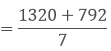= 301.171 cm2.

Therefore the area of the base is 301.71 cm2.

Question: 6

Find the curved surface area of a cone with base radius 5.25 cm and slant height 10 cm.

Solution:

It is given that:

Base radius of the cone (r) = 5.25 cm

Slant height of the cone (l) = 10 cm

Curved Surface Area (C. S. A) = πrl

= 22/7 * 5.25 * 10 = 165 cm2.

Therefore the curved surface area of the cone is 165 cm2

Question: 7

Find the total surface area of a cone, if its slant height is 21 m and diameter of its base is 24 m.

Solution:

It is given that:

Diameter of the cone (d) = 24 m

Radius of the cone(r) = 24/2 = 12 m

Slant height of the cone (l) = 21 m

T. S. A = Curved Surface Area of Cone + Area of Circular Base

= π ∗ r ∗ l + π ∗ r2

= (22/7 ∗ 12 ∗ 21) + (22/7 ∗ 12 ∗ 12) = 22/7 ∗ 12(12 + 21) = 1244.57 m2.

Therefore the total surface area of the cone is 1244.57 m2.

Question: 8

The area of the curved surface of a cone is 60 π cm2. If the slant height of the cone be 8 cm, find the radius of the base.

Solution:

It is given that:

Curved surface area (C. S. A) = 60 π cm2

Slant height of the cone (l) = 8 cm

Radius of the cone(r) = ?

Now we know,

Curved Surface Area (C. S. A) = πrl

⟹ πrl = 60 π cm2

⟹ r * 8 = 60

⟹ r = 7.5 cm

Therefore the radius of the base of the cone is 7.5 cm.

Question: 9

The curved surface area of a cone is 4070 cm2 and diameter is 70 cm .What is its slant height?

Solution:

Diameter of the cone (d) = 70 cm

Radius of the cone(r) = d2 = 35 cm

Slant height of the cone (l) = ?

Now,

Curved Surface Area = 4070 cm2

⟹ πrl = 4070

Where, r = Radius of the cone

l = Slant height of the cone

Therefore πrl = 4070

⟹ 22/7 ∗ 35 ∗ l = 4070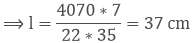Therefore slant height of the cone is 37 cm.

Question: 10

The radius and slant height of a cone are in the ratio 4: 7.If its curved surface area is 792 cm2, find its radius.

Solution:

It is given that

Curved surface area = πrl = 792

Let the radius (r) = 4x

Height (h) = 7x

Now,

C. S. A = 792

22/7 ∗ 4x ∗ 7x = 792

⟹ 88x2 = 792

⟹ x2 = 792/88 = 9

⟹ x = 3

Therefore Radius = 4x = 4 * 3 = 12 cm

Question: 11

A joker's cap is in the form of a right circular cone of base radius 7 cm and height 24 cm. Find the area of the sheet required to make 10 such caps.

Solution:

Given that,

Radius of conical cap(r) = 7 cm

Height of the conical cap (h) = 24 cm

Slant height (l) of conical cap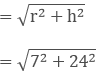= 25 cm

C.S.A of 1 conical cap = πrl

= 22/7 ∗ 7 ∗ 25

= 550 cm2

Curved surface area of 0 such conical caps = 5500 cm2

Thus, 5500 cm2 sheet will be required for making 10 caps.

Question: 12

Find the ratio of the curved surface area of two cones if their diameter of the bases are equal and slant heights are in the ratio 4: 3.

Solution:

Given that,

Diameter of two coins are equal.

Let r1 = r2 = r

Let ratio be x

Therefore slant height l1 of 1st cone = 4x

Similarly slant height l2 of 2nd cone = 3x

Therefore C. S. A1/C. S. A2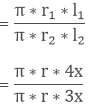= 4/3

Hence ratio is 4: 3

Question: 13

There are two cones .The curved surface area of one is twice that of the other. The slant height of the later is twice that of the former. Find the ratio of their radii.

Solution:

Let the curved surface area of 1st cone = 2x

C.S.A of 2nd cone = x

Slant height of 1st cone = h

Slant height of 2nd cone = 2h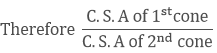= 2x/x

⟹ πr1l1/πr2l2 = 2/1

⟹ r1 ∗ h/r2 ∗ h = 2/1

⟹ r1/r2 = 4/1

Therefore ratio of r1 and r2 is 4:1.

Question: 14

The diameters of two cones are equal. If their slant height are in the ratio 5:4, find the ratio of their curved surfaces.

Solution:

It is given that

Diameters of two cones are equal

Therefore their radius are also equal i.e

r1 = r2

Let the ratio of slant height be x

Therefore l1 = 5x

l2 = 4x

Therefore Ratio of curved surface area = c1/c2

⟹ c1/c2 = πr1l1/πr2l2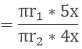= 5/4

∴ Ratio of curved surface area is 5: 4.

Question: 15

Curved surface area of a cone is 308 cm2 and its slant height is 14 cm. Find the radius of the base and total surface area of the cone.

Solution:

(1) It is given that

Slant height of cone = 14 cm

Let radius of circular end of cone = r

Curved surface area of cone = πrl

⟹ 308 cm2 = 22/7 ∗ r ∗ 14

⟹ r = 308/44 = 7 cm

Thus radius of circular end of cone = 7 cm.

(ii) It is given that C.S.A = 308 cm2

We know that total surface area of a cone

= curved surface area of a cone + Area of base

= πrl + πr2

= [308 + (22/7 ∗ 72)]

= 308 + 154

= 462 cm2

Thus total surface area of the cone is 462 cm2

Question: 16

The slant height and base diameter of a conical tomb are 25 m and 14 m respectively. Find the cost of white washing its curved surface area at the rate of Rs 210 per 100 m2.

Solution:

It is given that

Slant height of conical tomb (l) = 25 m

Base radius (r) of tomb = 14/2 = 7m

Curved surface area of conical length tomb = πrl

= 22/7 ∗ 7 ∗ 25

= 550 m2

Cost of white washing 100 m2 area = Rs 210Therefore the cost of white washing the whole tomb is Rs 1155.

Question: 17

A conical tent is 10 m high and the radius of it base is 24 m. Find the slant height of the tent. If the cost of 1 m2 canvas is Rs 70, find the cost of canvas required for the tent.

Solution:

It is given that

Height of the conical tent (h) = 10 m

Radius of conical tent (r) = 24 m

Let slant height of conical tent be l

l2 = h2 + r2

= 102 + 242 = 100 + 576

= 676 m2

⟹ l = 26 m

Thus, the slant height of the conical tent is 26 m.

(ii) It is given that:

Slant height (l) = 26 m

C.S.A of tent = πrl

= 22/7 ∗ 24 ∗ 26

= 1378/7 m2

Cost of 1m2 canvas = Rs 70

Cost of 1378/7 m2 canvas = Rs 1378/7 * 70 = Rs 1, 37, 280

Thus the cost of canvas required to make the tent is Rs 1, 37, 280.

Question: 18

A tent is in the form of a right circular cylinder surmounted by a cone. The diameter of cylinder is 24 m. The height of the cylindrical portion is 11m while the vertex of the cone is 16 m above the ground. Find the area of the canvas required for the tent.

Solution:

It is given that

Diameter of cylinder = 24 m

= 24/2 = 12 cm

Also radius of cone = 12 m

Height of cylinder = 11 m

Height of cone = 16 - 11 = 5 m

Slant height of cone= 13 m

Therefore area of canvas required for the tent = πrl + 2πrh

= 22/7 [(12 ∗ 13) + (2 ∗ 12 ∗ 11)] = 490.286 + 829.714

= 1320 m2

Question: 19

A circus tent is cylindrical to a height of 3 m and conical above it. If its diameter is 105 m and the slant height of the conical portion is 53 m, calculate the length of the canvas 5 m wide to make the required tent.

Solution:

Given diameter = 105 m,

Radius = 105/2 m = 52.5 m

Therefore curved surface area of circus tent = πrl + 2πrh

= (22/7 ∗ 52.5 ∗ 53) + (2 ∗ 22/7 ∗ 52.5 ∗ 3)

= 8745 + 990 = 9735 m2

Therefore length of the canvas required for tent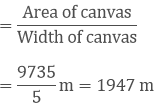Question: 20

The circumference of the base of a 10 m height conical tent is 44m, calculate the length of canvas used in making the tent if width of canvas is 2 m.

Solution:

We know that

C.S.A of cone = πrl

Given circumference = 2πr

⟹ 2 ∗ 227 ∗ r = 44

⟹ r/7 = 1

⟹ r = 7 m

Therefore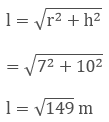Therefore C.S.A of tent = πrl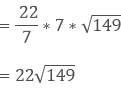Therefore the length of canvas used in making the tent= 134.2 m

Question: 21

What length of tarpaulin 4 m wide will be required to make a conical tent of height 8 m and base radius 6 m? Assume that the extra length of material will be required for stitching margins and wastage in cutting is approximately 20 cm.

Solution:

Given that,

Height of conical tent (h) = 8 m

Radius of base of tent (r) = 6 m

Slant height (l)C.S.A of conical tent = πrl

= (3.14 * 6 * 10) m2 = 188.4 m2

Let the length of tarpaulin sheet required be l

As 20 cm will wasted, so effective

Length will be (l - 0.2 m)

Area of sheet = C.S.A of sheet

[l * 0.2 * 3]m = 188.4 m2 = l - 0.2 m = 62.8 m

Accounting extra for wastage:

⟹ l = 63 m

Thus the length of the tarpaulin sheet will be = 63 m

Question: 22

A bus stop is barricaded from the remaining part of the road, by using 50 hollow cones made of recycled card board. Each cone has a base diameter of 40 cm and height 1m. If the outer side of each of the cones is to be painted and the cost of painting is Rs 12 per m2,what will be the cost of painting all these cones?

Solution:

The area to be painted is the curved surface area of each cone.

The formula of the curved surface area of a cone with base radius and slant height 7 is given as

Curved Surface Area = πrl

For each cone, we're given that the base diameter is 0.40 m.

Hence the base radius r = 0.20 m.

The vertical height l 1 = 1 m.

To find the slant height 'l' to be used in the formula for Curved Surface Area we use the following relation

Slant height,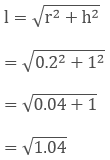l = 1.02 m

Now substituting the values of r = 0.2 m and slant height 1= 1.02 m and using pi = 3.14 in the formula of C.S.A.

We get Curved Surface Area = (3.14) (0.2) (1.02) = 0.64056 m2

This is the curved surface area of a single cone.

Since we need to paint 50 such cones the total area to be painted is,

Total area to be painted = (0.64056) (50) = 32.028 m2

The cost of painting is given as Rs. 12 per m2

Hence the total cost of painting = (12) (32.028) = 384.336

Hence, the total cost that would be incurred in the painting is Rs. 384.336

Question: 23

A cylinder and a cone have equal radii of their base and equal heights. If their curved surface area are in the ratio 8:5, show that the radius of each is to the height of each as 3:4.

Solution:

It is given that the base radius and the height of the cone and the cylinder are the same.

So let the base radius of each is 'r' and the vertical height of each is 'h'.

Let the slant height of the cone be 'l'.

The curved surface area of the cone = πrl

The curved surface area of the cylinder =

It is said that the ratio of the curved surface areas of the cylinder to that of the cone is 8:5

So,But we know thatSquaring on both sides we get:r2/h2 + 1 = 25/16

r2/h2 = 25/16 − 1

r2/h2 = 9/16

r/h = 3/4

Hence it is shown that the ratio of the radius to the height of the cone as well as the cylinder is: 3: 4

```### Course Features

• 728 Video Lectures
• Revision Notes
• Previous Year Papers
• Mind Map
• Study Planner
• NCERT Solutions
• Discussion Forum
• Test paper with Video Solution